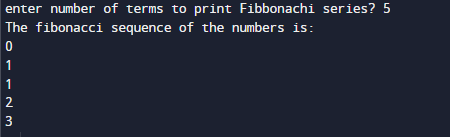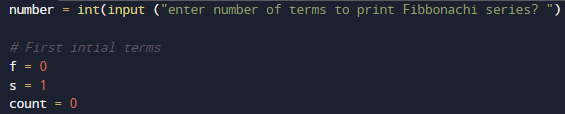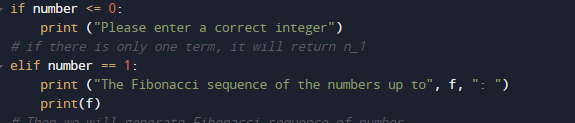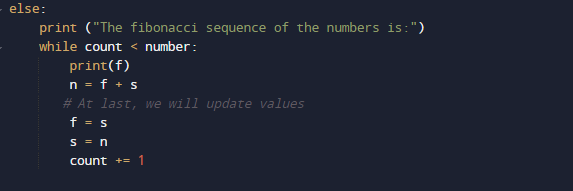# Python Program to Print the Fibonacci sequence

In this tutorial you will learn about the Python Program to Print the Fibonacci sequence and its application with practical example.

In this tutorial, we will learn to create a Python Program to Print the Fibonacci sequence using Python programming.

## Prerequisites

Before starting with this tutorial we assume that you are best aware of the following Pyhton programming topics:

• Basic Input and Output
• Basic Python  programming.
• Python if-else statements.
• Python Loop.

## Fibonacci sequence:

Take a look at Fibonacci sequence that begins with the 0 and 1 normally. 0, 1, 1, 2, 3, 5, 8, 13, 21, 34, 55, 89, 144, and so on . As you can see looking at  the series, you can easily understand the logic behind the series that each number in the sequence is the addition or sum of the two previous number.

## Python Program to Print the Fibonacci sequence

In this program we will find Fibonacci Sequence  between the given number using while loop. We would first declared and initialized the required variables. Next, we would prompt user to input a number . Later we will find Fibonacci sequence.

## OutputIn the above program, we have first declared and initialized a set variables required in the program.

• number= it will hold number value
• f= for holding first element value.
• s = for holding  second  elements value.
• n= it will hold next value.

After Declaring values we will take a number from user till we print the Fibonacci series of nth terms.and initiate f to zero (f=0) and s to one (s=1) and count to zero.and then we will check if the number of terms is valid or not ?If the given number is greater than 2, than within the while loop for finding the next term of Fibonacci sequence by adding the previous two terms.We will then update the variable by exchanging the value of f=s (second to first )and s=n (next to second )them, and it will continue till we will  reach the number of  terms the user wants to print.

In this tutorial we have learn about the Python Program to Print the Fibonacci sequence and its application with practical example. I hope you will like this tutorial.